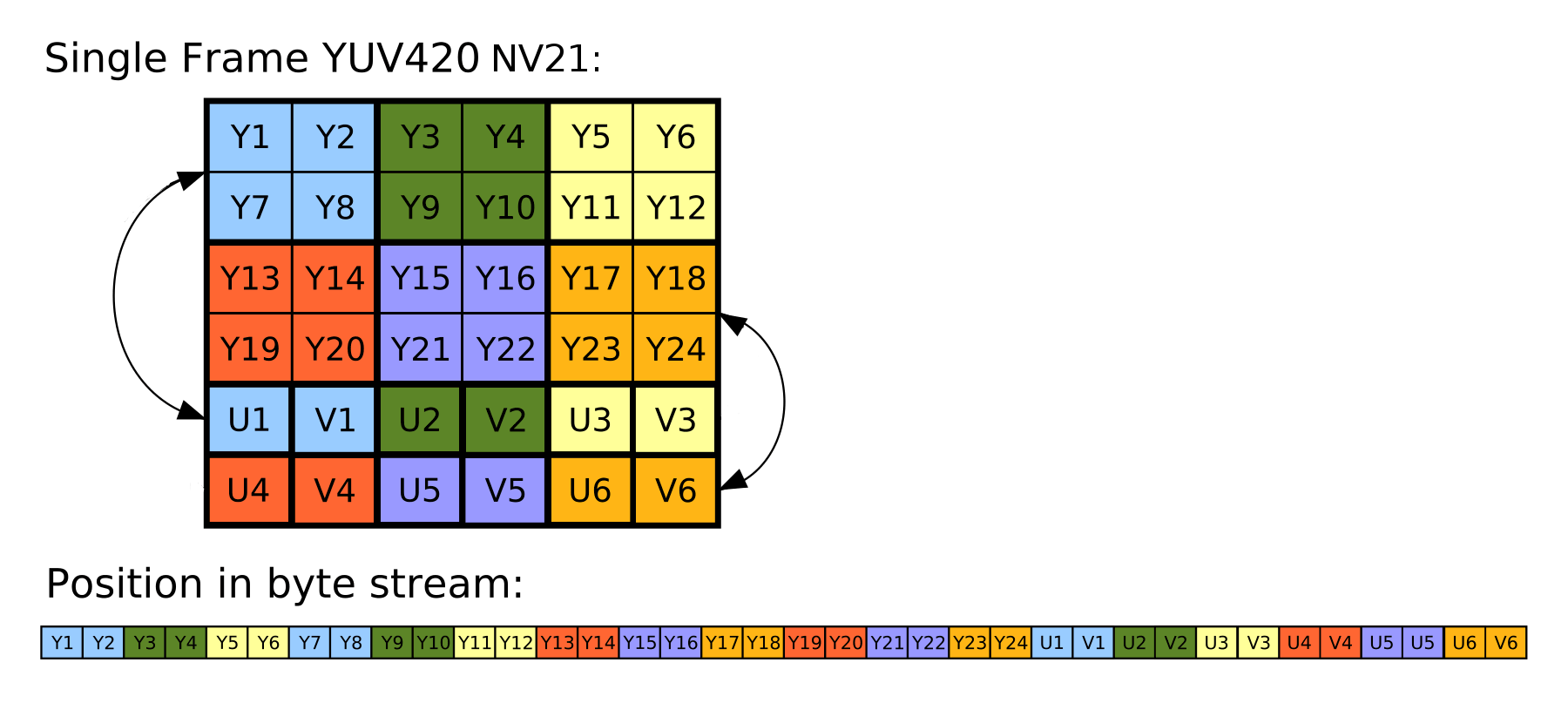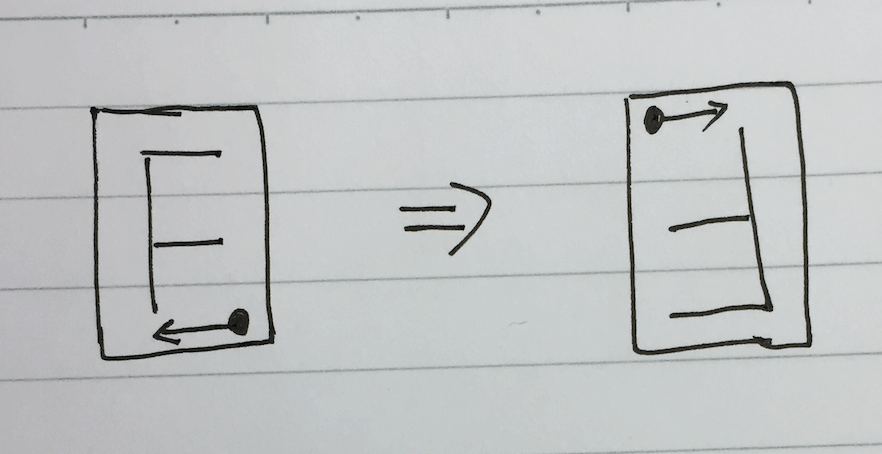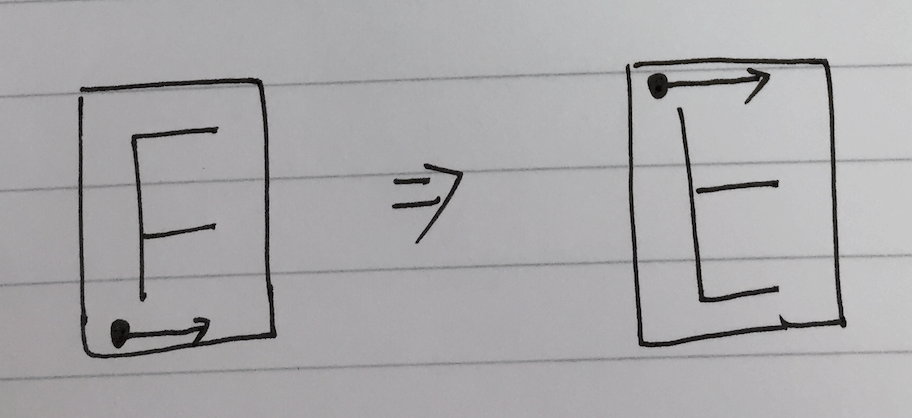# 记一次 YUV 图像翻转的性能优化

Posted by Piasy on May 24, 2017

## YUV 翻转

YUV 是一种图像编码格式（RGB 也是一种图像编码格式），YUV 主要用于视频，用来降低带宽。安卓平台上，用 Camera1 API 采集出来的数据（通过 `PreviewCallback` 回调获得）默认是 `YCbCr_420_SP`（简称 `NV21`，这是 YUV 的一种子格式）格式，NV21 的数据分布如下图所示（图片来源于 StackOverflow）：YUV 的操作其实都挺烧脑的，我每次都会画图来分析。``````private void flipImage(byte[] src, byte[] dst, int width, int height) {
int wh = width * height;

// Y
for (int i = 0; i < wh; i++) {
dst[i] = src[wh - i - 1];
}

// UV
for (int j = 0; j < wh / 2; j += 2) {
dst[wh + j] = src[wh + wh / 2 - j - 2];
dst[wh + j + 1] = src[wh + wh / 2 - j - 1];
}
}
````````````private void flipImageVertical(byte[] src, byte[] dst, int width, int height) {
// Y
for (int x = 0; x < width; x++) {
for (int y = 0; y < height; y++) {
dst[y * width + x] = src[(height - 1 - y) * width + x];
}
}

// UV
int wh = width * height;
int halfH = height / 2;
for (int x = 0; x < width; x += 2) {
for (int y = 0; y < halfH; y++) {
dst[wh + y * width + x] = src[wh + (halfH - 1 - y) * width + x];
dst[wh + y * width + x + 1] = src[wh + (halfH - 1 - y) * width + x + 1];
}
}
}
``````

## 性能优化

《Unix 编程艺术》中有一条优化原则：

### 优化思路一：native 化

Java 最大的特点就是简单，没有 C++ 那样海量的语言特性难以驾驭，但 Java 还有另一个特点就是性能差，同样的代码，C++ 版本的速度是 Java 版本的几倍甚至几十倍。所以遇到计算型任务的性能问题，首先想到的就是把计算转移到 native 层，这是最快的方法，往往也是效果最明显的方法。

### 优化思路二：乘除变加减

``````private void flipImageVertical(byte[] src, byte[] dst, int width, int height) {
// Y
int endIdx = (height - 1) * width;
for (int x = 0; x < width; x++) {
int dstIdx = x;
int srcIdx = endIdx + x;
for (int y = 0; y < height; y++) {
dst[dstIdx] = src[srcIdx];
dstIdx += width;
srcIdx -= width;
}
}

// UV
int wh = width * height;
int halfH = height / 2;
endIdx = (halfH - 1) * width;
for (int x = 0; x < width; x += 2) {
int dstIdx = wh + x;
int srcIdx = wh + endIdx + x;
for (int y = 0; y < halfH; y++) {
dst[dstIdx] = src[srcIdx];
dst[dstIdx + 1] = src[srcIdx + 1];
dstIdx += width;
srcIdx -= width;
}
}
}
``````

### 优化思路三：内存访问模式

``````private void flipImageVertical(byte[] src, byte[] dst, int width, int height) {
// Y
for (int y = 0; y < height; y++) {
System.arraycopy(src, (height - 1 - y) * width, dst, y * width, width);
}

// UV
int wh = width * height;
int halfH = height / 2;
for (int y = 0; y < halfH; y++) {
System.arraycopy(src, wh + y * width, dst, wh + (halfH - 1 - y) * width, width);
}
}
``````

## 后记